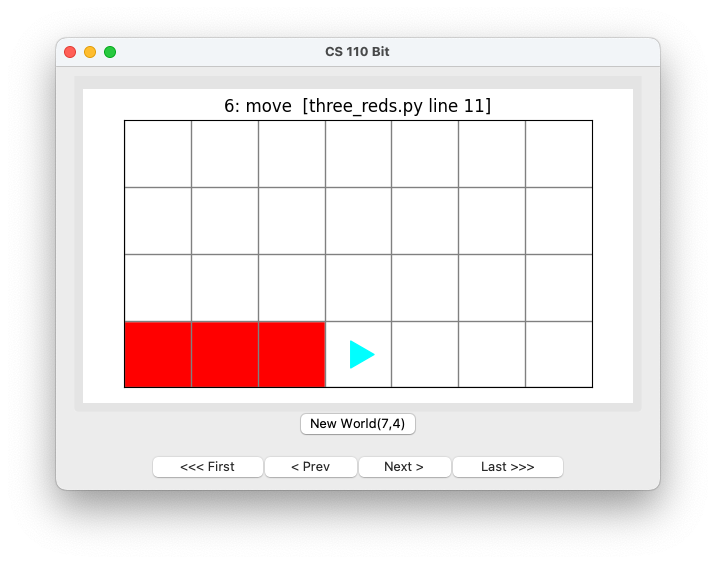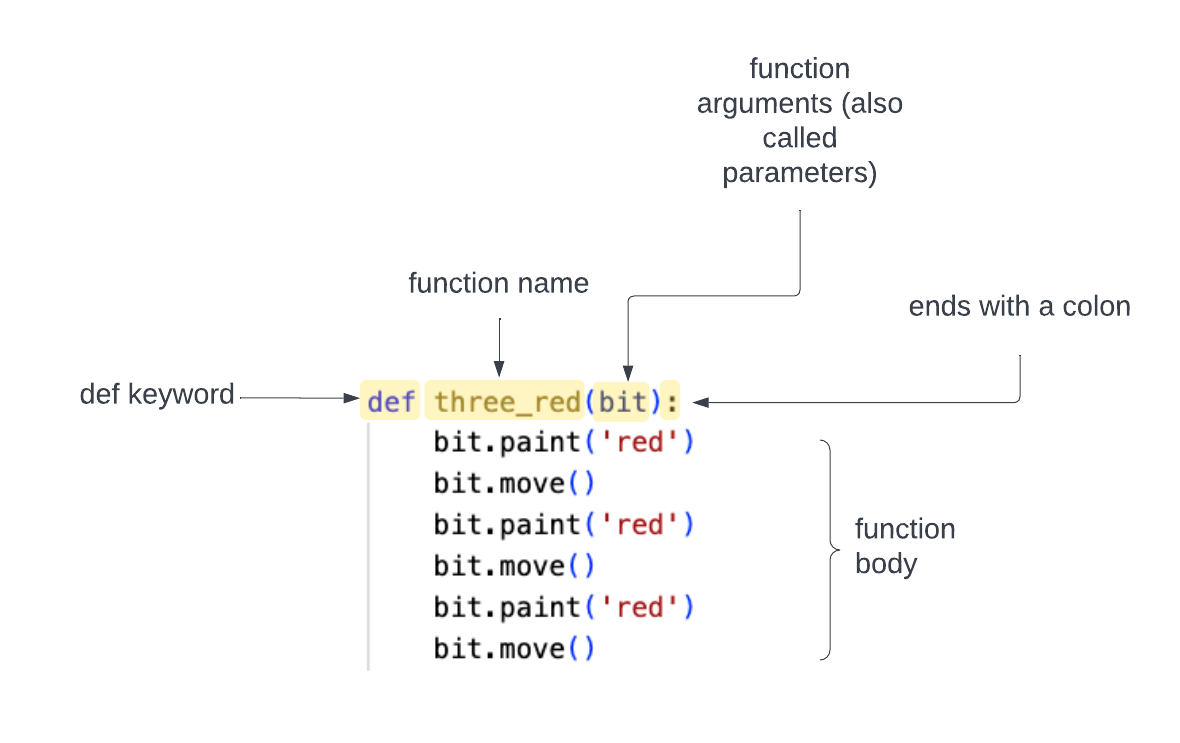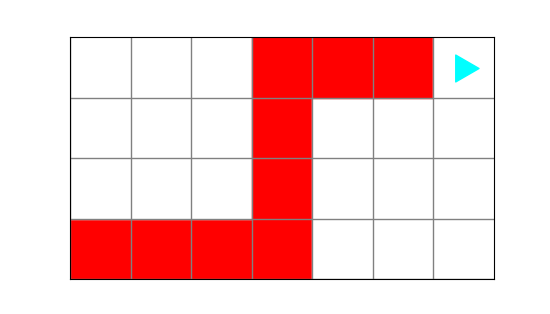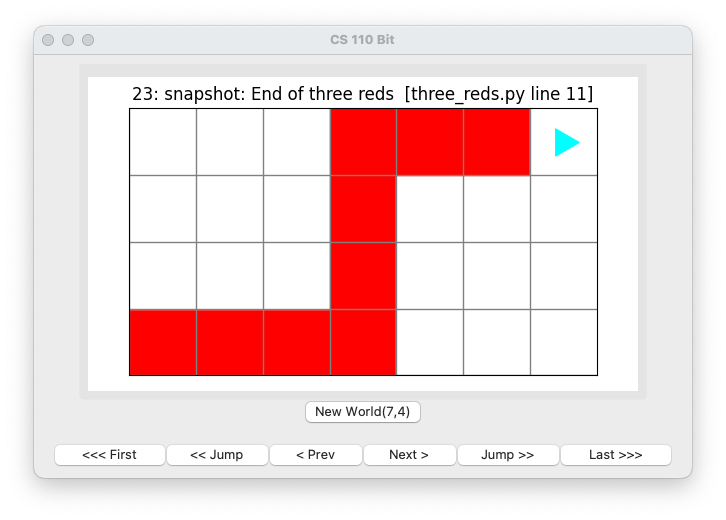Computer Science

# Functions

In your `bit` folder, create a file called `three_reds.py` and put this code in it:

``````from byubit import Bit

@Bit.empty_world(7, 4)
def run(bit):
bit.paint('red')
bit.move()
bit.paint('red')
bit.move()
bit.paint('red')
bit.move()

if __name__ == '__main__':
run(Bit.new_bit)``````

If you run this code, you will see that Bit draws three red squares:But what if you wanted to paint three red squares lots of times? If you want to do this, it helps a lot to define your own functions. You could write a function called `three_red()` that paints three red squares:

``````def three_red(bit):
bit.paint('red')
bit.move()
bit.paint('red')
bit.move()
bit.paint('red')
bit.move()``````

There are five parts to a function:1. The `def keyword` tells Python you are starting a function definition.

2. The `function name` tells Python the name of the function.

3. The `function arguments (or parameters)` tell Python what information the function uses when it runs (in this case uses bit) and must be in parentheses.

4. The `colon` ends the first line of the function.

5. The `function body` is indented four spaces and contains the instructions that will run when the function is called.

For now, we will always have one argument to your functions — `bit`. In the future, we will show you how to use multiple arguments.

You can call this function in your code by typing:

``three_red(bit)``

Replace the contents of your `three_reds.py` file with:

``````from byubit import Bit

def three_red(bit):
bit.paint('red')
bit.move()
bit.paint('red')
bit.move()
bit.paint('red')
bit.move()

@Bit.empty_world(7, 4)
def run(bit):
three_red(bit)
bit.left()
three_red(bit)
bit.right()
three_red(bit)

if __name__ == '__main__':
run(Bit.new_bit)``````

Here we call `three_red()`, turn left, call `three_red()` again, turn right, and then call `three_red()` again.If you run this code, you should see:## The Bit decorator

Look at the code above. Notice that the decorator — `@Bit.empty_world(7, 4)` — only goes on the `run()` function. It is not on top of our new `three_red()` function.

You should only put the Bit decorator on your very first function — which is usually the only function called in side of your main block.

## Snapshots

As soon as you start writing larger Bit programs, you will find it becomes tedious to click the `Next` and `Prev` buttons to see what your program is doing. To make things simpler, you can take a snapshot of your code at any point:

``bit.snapshot('some descriptive name')``

Modify your `three_reds.py` file by adding one line to the end of your `three_red()` function:

``````def three_red(bit):
bit.paint('red')
bit.move()
bit.paint('red')
bit.move()
bit.paint('red')
bit.move()
bit.snapshot('End of three reds')``````

Now when you run this program, Bit will create a snapshot every time it finishes running `three_red()`. You will see a new buttons called `Jump`:Pressing these will jump to the previous or next snapshot. By creating a snapshot at the end of the function, this lets you jump through your program one function call at a time.

## Calling a function

Let’s look at calling a function one more time. In your `bit` directory, create a file called `coloring_squares.py` and put this code in it:

``````from byubit import Bit

def reds(bit):
bit.paint('red')
bit.move()
bit.paint('red')
bit.move()

def blues(bit):
bit.paint('blue')
bit.move()
bit.paint('blue')
bit.move()

def greens(bit):
bit.paint('green')
bit.move()
bit.paint('green')
bit.move()

@Bit.empty_world(7, 3)
def run(bit):
reds(bit)
greens(bit)

if __name__ == '__main__':
run(Bit.new_bit)``````

Take a look at this program. What do you think it will do?If you run the program, it creates this world:Why didn’t it paint anything blue?

Remember, functions need to be called in order to run.We defined the `blues()` function, but we never called it. Since we never called it, `blues()` never runs, so no blue squares are ever painted.

## Docstrings

When you write a function in Python, you can add a docstring to provide helpful comments on what the function does. For example, when writing our `greens()` function above, we could have written:

``````def greens(bit):
"""
Colors two squares green, starting with the current square.

Bit ends on the third square, facing in the same direction as it started.
"""
bit.paint('green')
bit.move()
bit.paint('green')
bit.move()``````

A docstring starts and ends with triple quotes `"""`.

A good docstring will describe what the function does and tell you the starting and ending conditions. For example in the docstring above it tells you that it starts on the current square and ends on the third square, facing in the same direction as it started. This kind of context can help you keep track of what each function does.

## Naming a function

When you name a function, follow these guidelines:

• Use lowercase letters
• Use underscores `_` to break up compound names. Your function should be called `move_around()` not `movearound()` or `moveAround()`.